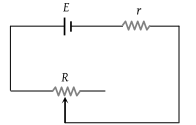# NEET Questions Solved

PMT - 1995

A battery of e.m.f. E and internal resistance r is connected to a variable resistor R as shown here. Which one of the following is true(1) Potential difference across the terminals of the battery is maximum when R = r

(2) Power delivered to the resistor is maximum when R = r

(3) Current in the circuit is maximum when R = r

(4) Current in the circuit is maximum when R >> r

(2) For power to be maximum

External resistance = Equivalent internal resistance of the circuit

Difficulty Level:

• 31%
• 35%
• 31%
• 6%